# Example: Mean off-time reduction in Parkinson’s disease

library(multinma)
options(mc.cores = parallel::detectCores())

This vignette describes the analysis of data on the mean off-time reduction in patients given dopamine agonists as adjunct therapy in Parkinson’s disease, in a network of 7 trials of 4 active drugs plus placebo (Dias et al. 2011). The data are available in this package as parkinsons:

head(parkinsons)
#>   studyn trtn     y    se   n  diff se_diff
#> 1      1    1 -1.22 0.504  54    NA   0.504
#> 2      1    3 -1.53 0.439  95 -0.31   0.668
#> 3      2    1 -0.70 0.282 172    NA   0.282
#> 4      2    2 -2.40 0.258 173 -1.70   0.382
#> 5      3    1 -0.30 0.505  76    NA   0.505
#> 6      3    2 -2.60 0.510  71 -2.30   0.718

We consider analysing these data in three separate ways:

1. Using arm-based data (means y and corresponding standard errors se);
2. Using contrast-based data (mean differences diff and corresponding standard errors se_diff);
3. A combination of the two, where some studies contribute arm-based data, and other contribute contrast-based data.

Note: In this case, with Normal likelihoods for both arms and contrasts, we will see that the three analyses give identical results. In general, unless the arm-based likelihood is Normal, results from a model using a contrast-based likelihood will not exactly match those from a model using an arm-based likelihood, since the contrast-based Normal likelihood is only an approximation. Similarity of results depends on the suitability of the Normal approximation, which may not always be appropriate - e.g. with a small number of events or small sample size for a binary outcome. The use of an arm-based likelihood (sometimes called an “exact” likelihood) is therefore preferable where possible in general.

## Analysis of arm-based data

We begin with an analysis of the arm-based data - means and standard errors.

### Setting up the network

We have arm-level continuous data giving the mean off-time reduction (y) and standard error (se) in each arm. We use the function set_agd_arm() to set up the network.

arm_net <- set_agd_arm(parkinsons,
study = studyn,
trt = trtn,
y = y,
se = se,
sample_size = n)
arm_net
#> A network with 7 AgD studies (arm-based).
#>
#> ------------------------------------------------------- AgD studies (arm-based) ----
#>  Study Treatments
#>  1     2: 1 | 3
#>  2     2: 1 | 2
#>  3     3: 1 | 2 | 4
#>  4     2: 3 | 4
#>  5     2: 3 | 4
#>  6     2: 4 | 5
#>  7     2: 4 | 5
#>
#>  Outcome type: continuous
#> ------------------------------------------------------------------------------------
#> Total number of treatments: 5
#> Total number of studies: 7
#> Reference treatment is: 4
#> Network is connected

We let treatment 4 be set by default as the network reference treatment, since this results in considerably improved sampling efficiency over choosing treatment 1 as the network reference. The sample_size argument is optional, but enables the nodes to be weighted by sample size in the network plot.

Plot the network structure.

plot(arm_net, weight_edges = TRUE, weight_nodes = TRUE)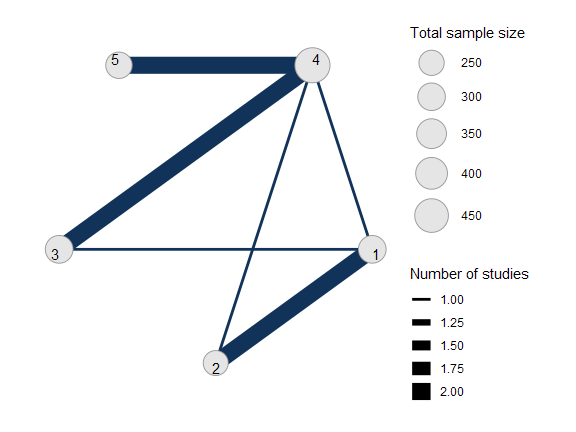### Meta-analysis models

We fit both fixed effect (FE) and random effects (RE) models.

#### Fixed effect meta-analysis

First, we fit a fixed effect model using the nma() function with trt_effects = "fixed". We use $$\mathrm{N}(0, 100^2)$$ prior distributions for the treatment effects $$d_k$$ and study-specific intercepts $$\mu_j$$. We can examine the range of parameter values implied by these prior distributions with the summary() method:

summary(normal(scale = 100))
#> A Normal prior distribution: location = 0, scale = 100.
#> 50% of the prior density lies between -67.45 and 67.45.
#> 95% of the prior density lies between -196 and 196.

The model is fitted using the nma() function.

arm_fit_FE <- nma(arm_net,
trt_effects = "fixed",
prior_intercept = normal(scale = 100),
prior_trt = normal(scale = 10))
#> Note: Setting "4" as the network reference treatment.

Basic parameter summaries are given by the print() method:

arm_fit_FE
#> A fixed effects NMA with a normal likelihood (identity link).
#> Inference for Stan model: normal.
#> 4 chains, each with iter=2000; warmup=1000; thin=1;
#> post-warmup draws per chain=1000, total post-warmup draws=4000.
#>
#>       mean se_mean   sd   2.5%   25%   50%   75% 97.5% n_eff Rhat
#> d  0.53    0.01 0.48  -0.38  0.21  0.53  0.85  1.50  1681    1
#> d -1.27    0.01 0.52  -2.28 -1.62 -1.29 -0.91 -0.24  1760    1
#> d  0.05    0.01 0.31  -0.57 -0.16  0.05  0.25  0.66  2363    1
#> d -0.30    0.00 0.21  -0.72 -0.44 -0.29 -0.15  0.11  2793    1
#> lp__ -6.70    0.06 2.39 -12.28 -8.08 -6.38 -4.96 -3.07  1579    1
#>
#> Samples were drawn using NUTS(diag_e) at Tue Jun 23 15:49:17 2020.
#> For each parameter, n_eff is a crude measure of effective sample size,
#> and Rhat is the potential scale reduction factor on split chains (at
#> convergence, Rhat=1).

By default, summaries of the study-specific intercepts $$\mu_j$$ are hidden, but could be examined by changing the pars argument:

# Not run
print(arm_fit_FE, pars = c("d", "mu"))

The prior and posterior distributions can be compared visually using the plot_prior_posterior() function:

plot_prior_posterior(arm_fit_FE)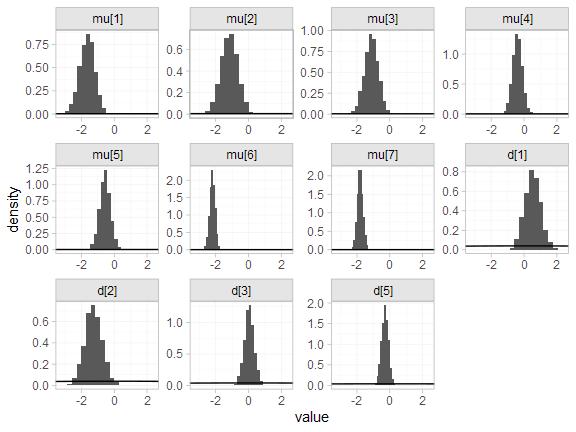#### Random effects meta-analysis

We now fit a random effects model using the nma() function with trt_effects = "random". Again, we use $$\mathrm{N}(0, 100^2)$$ prior distributions for the treatment effects $$d_k$$ and study-specific intercepts $$\mu_j$$, and we additionally use a $$\textrm{half-N}(5^2)$$ prior for the heterogeneity standard deviation $$\tau$$. We can examine the range of parameter values implied by these prior distributions with the summary() method:

summary(normal(scale = 100))
#> A Normal prior distribution: location = 0, scale = 100.
#> 50% of the prior density lies between -67.45 and 67.45.
#> 95% of the prior density lies between -196 and 196.
summary(half_normal(scale = 5))
#> A half-Normal prior distribution: location = 0, scale = 5.
#> 50% of the prior density lies between 0 and 3.37.
#> 95% of the prior density lies between 0 and 9.8.

Fitting the RE model

arm_fit_RE <- nma(arm_net,
seed = 379394727,
trt_effects = "random",
prior_intercept = normal(scale = 100),
prior_trt = normal(scale = 100),
prior_het = half_normal(scale = 5),
#> Note: Setting "4" as the network reference treatment.
#> Warning: There were 2 divergent transitions after warmup. Increasing adapt_delta above 0.99 may help. See
#> http://mc-stan.org/misc/warnings.html#divergent-transitions-after-warmup
#> Warning: Examine the pairs() plot to diagnose sampling problems

We do see a small number of divergent transition errors, which cannot simply be removed by increasing the value of the adapt_delta argument (by default set to 0.95 for RE models). To diagnose, we use the pairs() method to investigate where in the posterior distribution these divergences are happening (indicated by red crosses):

pairs(arm_fit_RE, pars = c("mu", "d", "delta[4: 3]", "tau"))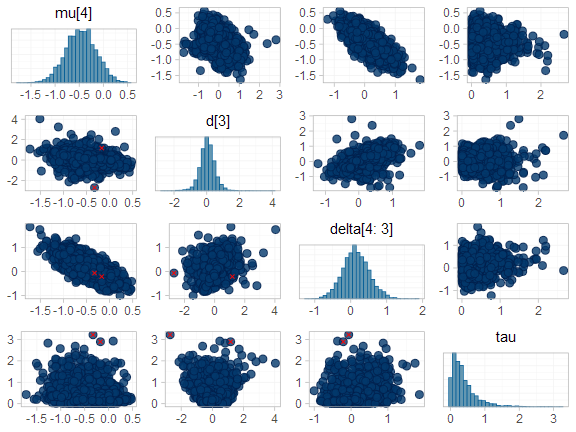The divergent transitions occur in the upper tail of the heterogeneity standard deviation. In this case, with only a small number of studies, there is not very much information to estimate the heterogeneity standard deviation and the prior distribution may be too heavy-tailed. We could consider a more informative prior distribution for the heterogeneity variance to aid estimation.

Basic parameter summaries are given by the print() method:

arm_fit_RE
#> A random effects NMA with a normal likelihood (identity link).
#> Inference for Stan model: normal.
#> 4 chains, each with iter=2000; warmup=1000; thin=1;
#> post-warmup draws per chain=1000, total post-warmup draws=4000.
#>
#>        mean se_mean   sd   2.5%    25%    50%    75% 97.5% n_eff Rhat
#> d   0.54    0.02 0.65  -0.67   0.14   0.54   0.92  1.81  1551 1.00
#> d  -1.29    0.02 0.72  -2.74  -1.71  -1.28  -0.88  0.17  1619 1.00
#> d   0.04    0.01 0.48  -0.89  -0.23   0.04   0.31  0.96  2038 1.00
#> d  -0.29    0.01 0.44  -1.16  -0.50  -0.29  -0.09  0.59  2238 1.00
#> lp__ -12.88    0.10 3.59 -20.79 -15.15 -12.59 -10.31 -6.82  1353 1.01
#> tau    0.39    0.01 0.38   0.01   0.13   0.29   0.53  1.42   711 1.01
#>
#> Samples were drawn using NUTS(diag_e) at Tue Jun 23 15:49:36 2020.
#> For each parameter, n_eff is a crude measure of effective sample size,
#> and Rhat is the potential scale reduction factor on split chains (at
#> convergence, Rhat=1).

By default, summaries of the study-specific intercepts $$\mu_j$$ and study-specific relative effects $$\delta_{jk}$$ are hidden, but could be examined by changing the pars argument:

# Not run
print(arm_fit_RE, pars = c("d", "mu", "delta"))

The prior and posterior distributions can be compared visually using the plot_prior_posterior() function:

plot_prior_posterior(arm_fit_RE)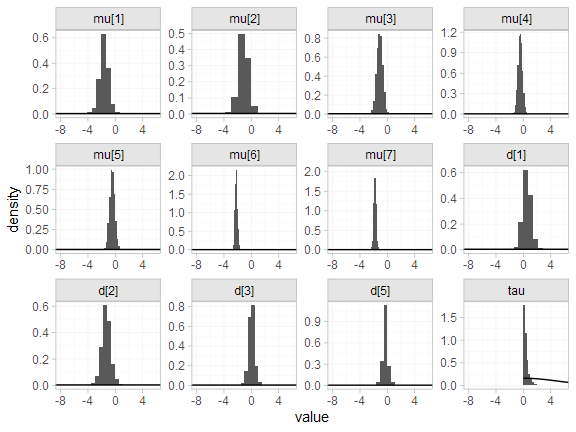#### Model comparison

Model fit can be checked using the dic() function:

(arm_dic_FE <- dic(arm_fit_FE))
#> Residual deviance: 13.4 (on 15 data points)
#>                pD: 11.1
#>               DIC: 24.5
(arm_dic_RE <- dic(arm_fit_RE))
#> Residual deviance: 13.8 (on 15 data points)
#>                pD: 12.7
#>               DIC: 26.5

Both models fit the data well, having posterior mean residual deviance close to the number of data points. The DIC is similar between models, so we choose the FE model based on parsimony.

We can also examine the residual deviance contributions with the corresponding plot() method.

plot(arm_dic_FE)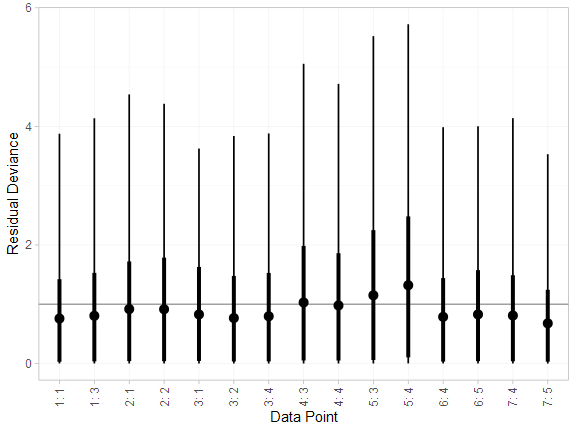plot(arm_dic_RE)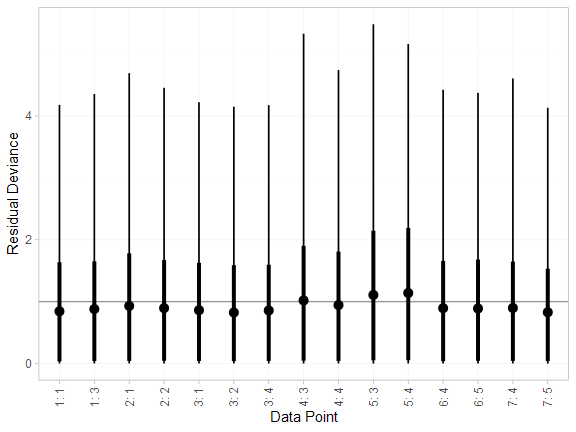### Further results

For comparison with Dias et al. (2011), we can produce relative effects against placebo using the relative_effects() function with trt_ref = 1:

(arm_releff_FE <- relative_effects(arm_fit_FE, trt_ref = 1))
#>       mean   sd  2.5%   25%   50%   75% 97.5% Bulk_ESS Tail_ESS Rhat
#> d -0.53 0.48 -1.50 -0.85 -0.53 -0.21  0.38     1697     2009    1
#> d -1.81 0.34 -2.46 -2.04 -1.80 -1.57 -1.11     5164     3060    1
#> d -0.49 0.49 -1.45 -0.82 -0.48 -0.14  0.47     2369     2543    1
#> d -0.83 0.53 -1.88 -1.18 -0.82 -0.47  0.17     1766     2337    1
plot(arm_releff_FE, ref_line = 0)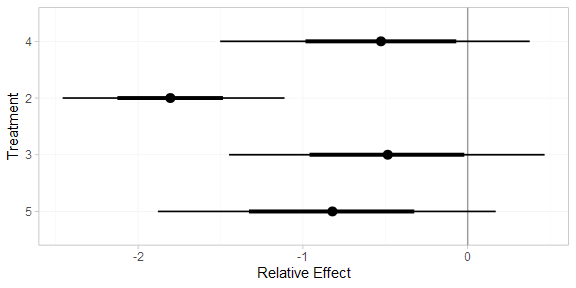(arm_releff_RE <- relative_effects(arm_fit_RE, trt_ref = 1))
#>       mean   sd  2.5%   25%   50%   75% 97.5% Bulk_ESS Tail_ESS Rhat
#> d -0.54 0.65 -1.81 -0.92 -0.54 -0.14  0.67     1728     1513    1
#> d -1.83 0.53 -2.92 -2.10 -1.83 -1.54 -0.83     3878     2438    1
#> d -0.50 0.65 -1.79 -0.89 -0.48 -0.11  0.71     2372     2138    1
#> d -0.83 0.77 -2.35 -1.27 -0.82 -0.41  0.66     1913     1653    1
plot(arm_releff_RE, ref_line = 0)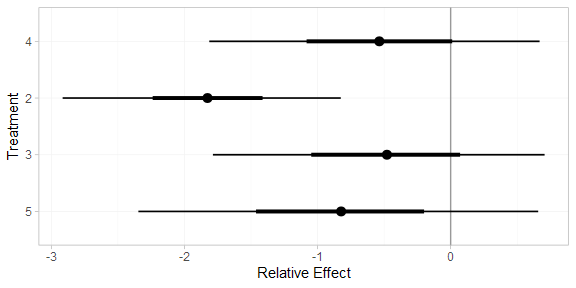Following Dias et al. (2011), we produce absolute predictions of the mean off-time reduction on each treatment assuming a Normal distribution for the outcomes on treatment 1 (placebo) with mean $$-0.73$$ and precision $$21$$. We use the predict() method, where the baseline argument takes a distr() distribution object with which we specify the corresponding Normal distribution, and we specify trt_ref = 1 to indicate that the baseline distribution corresponds to treatment 1. (Strictly speaking, type = "response" is unnecessary here, since the identity link function was used.)

arm_pred_FE <- predict(arm_fit_FE,
baseline = distr(qnorm, mean = -0.73, sd = 21^-0.5),
type = "response",
trt_ref = 1)
arm_pred_FE
#>          mean   sd  2.5%   25%   50%   75% 97.5% Bulk_ESS Tail_ESS Rhat
#> pred -1.26 0.52 -2.31 -1.60 -1.26 -0.90 -0.25     1895     2221    1
#> pred -0.73 0.22 -1.16 -0.88 -0.73 -0.58 -0.29     3856     3973    1
#> pred -2.53 0.41 -3.31 -2.81 -2.54 -2.26 -1.74     4715     3448    1
#> pred -1.22 0.53 -2.29 -1.57 -1.21 -0.85 -0.17     2526     2443    1
#> pred -1.56 0.57 -2.70 -1.93 -1.56 -1.18 -0.48     1963     2503    1
plot(arm_pred_FE)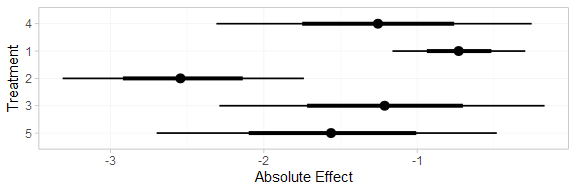arm_pred_RE <- predict(arm_fit_RE,
baseline = distr(qnorm, mean = -0.73, sd = 21^-0.5),
type = "response",
trt_ref = 1)
arm_pred_RE
#>          mean   sd  2.5%   25%   50%   75% 97.5% Bulk_ESS Tail_ESS Rhat
#> pred -1.27 0.68 -2.58 -1.67 -1.26 -0.84  0.02     1855     1799    1
#> pred -0.73 0.22 -1.16 -0.87 -0.73 -0.58 -0.30     4018     3891    1
#> pred -2.56 0.57 -3.71 -2.87 -2.56 -2.22 -1.46     4310     2575    1
#> pred -1.23 0.69 -2.63 -1.64 -1.21 -0.80  0.06     2544     2354    1
#> pred -1.56 0.80 -3.12 -2.03 -1.56 -1.09 -0.04     2013     1955    1
plot(arm_pred_RE)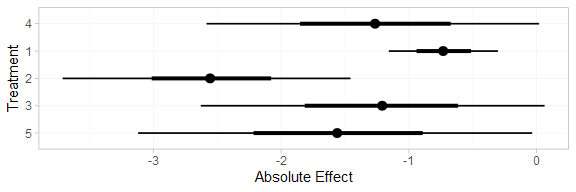If the baseline argument is omitted, predictions of mean off-time reduction will be produced for every study in the network based on their estimated baseline response $$\mu_j$$:

arm_pred_FE_studies <- predict(arm_fit_FE, type = "response")
arm_pred_FE_studies
#> ---------------------------------------------------------------------- Study: 1 ----
#>
#>             mean   sd  2.5%   25%   50%   75% 97.5% Bulk_ESS Tail_ESS Rhat
#> pred[1: 4] -1.65 0.45 -2.56 -1.95 -1.65 -1.35 -0.78     2204     2569    1
#> pred[1: 1] -1.12 0.43 -1.94 -1.40 -1.13 -0.83 -0.27     3520     3571    1
#> pred[1: 2] -2.92 0.52 -3.92 -3.28 -2.93 -2.58 -1.89     3615     3032    1
#> pred[1: 3] -1.61 0.39 -2.38 -1.87 -1.60 -1.34 -0.86     3713     2971    1
#> pred[1: 5] -1.95 0.50 -2.95 -2.28 -1.93 -1.60 -1.01     2115     2346    1
#>
#> ---------------------------------------------------------------------- Study: 2 ----
#>
#>             mean   sd  2.5%   25%   50%   75% 97.5% Bulk_ESS Tail_ESS Rhat
#> pred[2: 4] -1.18 0.51 -2.20 -1.52 -1.17 -0.83 -0.23     1696     1706    1
#> pred[2: 1] -0.65 0.26 -1.16 -0.82 -0.65 -0.47 -0.13     4865     3295    1
#> pred[2: 2] -2.45 0.24 -2.92 -2.61 -2.45 -2.29 -1.97     4559     3225    1
#> pred[2: 3] -1.13 0.53 -2.17 -1.49 -1.12 -0.77 -0.11     2377     2114    1
#> pred[2: 5] -1.48 0.56 -2.57 -1.86 -1.47 -1.09 -0.41     1742     2217    1
#>
#> ---------------------------------------------------------------------- Study: 3 ----
#>
#>             mean   sd  2.5%   25%   50%   75% 97.5% Bulk_ESS Tail_ESS Rhat
#> pred[3: 4] -1.12 0.42 -1.94 -1.39 -1.11 -0.83 -0.31     2042     2678    1
#> pred[3: 1] -0.58 0.36 -1.26 -0.83 -0.58 -0.34  0.14     4391     3519    1
#> pred[3: 2] -2.39 0.39 -3.17 -2.65 -2.40 -2.11 -1.61     4301     2814    1
#> pred[3: 3] -1.07 0.47 -2.01 -1.38 -1.07 -0.76 -0.17     3045     2738    1
#> pred[3: 5] -1.41 0.47 -2.35 -1.73 -1.40 -1.09 -0.53     2084     2701    1
#>
#> ---------------------------------------------------------------------- Study: 4 ----
#>
#>             mean   sd  2.5%   25%   50%   75% 97.5% Bulk_ESS Tail_ESS Rhat
#> pred[4: 4] -0.39 0.29 -0.98 -0.59 -0.39 -0.20  0.17     2620     3104    1
#> pred[4: 1]  0.14 0.51 -0.86 -0.22  0.14  0.48  1.19     2237     2354    1
#> pred[4: 2] -1.67 0.56 -2.73 -2.06 -1.68 -1.29 -0.55     2302     2049    1
#> pred[4: 3] -0.35 0.25 -0.82 -0.51 -0.35 -0.18  0.14     5259     3307    1
#> pred[4: 5] -0.69 0.36 -1.41 -0.93 -0.68 -0.45  0.01     2374     2634    1
#>
#> ---------------------------------------------------------------------- Study: 5 ----
#>
#>             mean   sd  2.5%   25%   50%   75% 97.5% Bulk_ESS Tail_ESS Rhat
#> pred[5: 4] -0.57 0.33 -1.24 -0.78 -0.56 -0.35  0.07     3062     3078    1
#> pred[5: 1] -0.03 0.53 -1.06 -0.39 -0.03  0.32  1.00     2268     2373    1
#> pred[5: 2] -1.84 0.59 -3.00 -2.22 -1.85 -1.47 -0.67     2300     2269    1
#> pred[5: 3] -0.52 0.29 -1.10 -0.71 -0.52 -0.32  0.06     4927     3499    1
#> pred[5: 5] -0.86 0.39 -1.65 -1.12 -0.85 -0.60 -0.12     2773     2678    1
#>
#> ---------------------------------------------------------------------- Study: 6 ----
#>
#>             mean   sd  2.5%   25%   50%   75% 97.5% Bulk_ESS Tail_ESS Rhat
#> pred[6: 4] -2.20 0.17 -2.56 -2.32 -2.20 -2.08 -1.87     3067     2596    1
#> pred[6: 1] -1.67 0.51 -2.63 -2.03 -1.68 -1.32 -0.64     1771     2353    1
#> pred[6: 2] -3.47 0.55 -4.54 -3.86 -3.47 -3.10 -2.37     1807     2282    1
#> pred[6: 3] -2.16 0.36 -2.84 -2.40 -2.16 -1.91 -1.45     2284     2480    1
#> pred[6: 5] -2.50 0.17 -2.84 -2.61 -2.50 -2.38 -2.16     6118     2868    1
#>
#> ---------------------------------------------------------------------- Study: 7 ----
#>
#>             mean   sd  2.5%   25%   50%   75% 97.5% Bulk_ESS Tail_ESS Rhat
#> pred[7: 4] -1.80 0.18 -2.14 -1.92 -1.81 -1.69 -1.43     3275     2504    1
#> pred[7: 1] -1.27 0.51 -2.27 -1.62 -1.28 -0.93 -0.24     1728     2246    1
#> pred[7: 2] -3.07 0.56 -4.14 -3.44 -3.08 -2.69 -1.97     1795     2353    1
#> pred[7: 3] -1.76 0.36 -2.46 -1.99 -1.77 -1.51 -1.02     2380     2584    1
#> pred[7: 5] -2.10 0.21 -2.52 -2.24 -2.09 -1.96 -1.70     4516     3023    1
plot(arm_pred_FE_studies)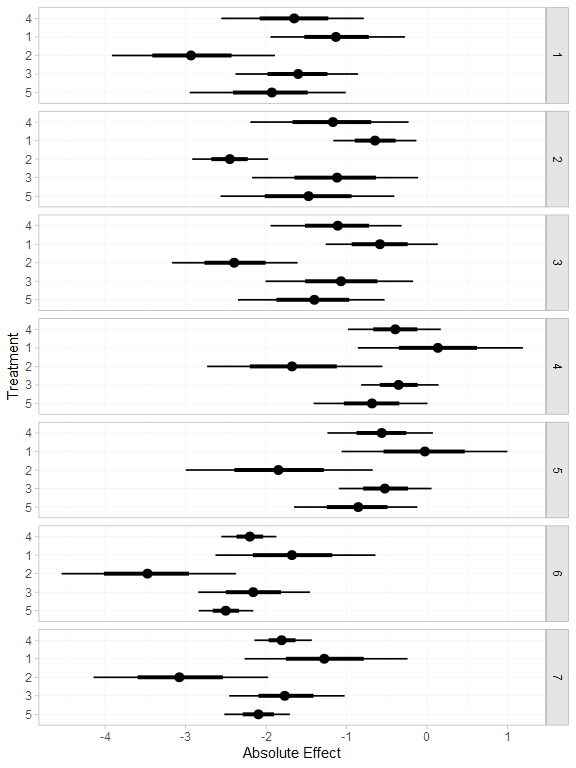We can also produce treatment rankings, rank probabilities, and cumulative rank probabilities.

(arm_ranks <- posterior_ranks(arm_fit_FE))
#>         mean   sd 2.5% 25% 50% 75% 97.5% Bulk_ESS Tail_ESS Rhat
#> rank 3.50 0.71    2   3   3   4     5     2288       NA    1
#> rank 4.64 0.77    2   5   5   5     5     2306       NA    1
#> rank 1.06 0.31    1   1   1   1     2     2086     2190    1
#> rank 3.53 0.92    2   3   4   4     5     2819       NA    1
#> rank 2.27 0.68    1   2   2   2     4     2507     2622    1
plot(arm_ranks)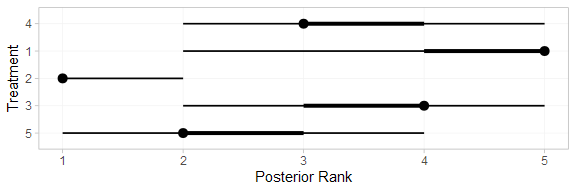(arm_rankprobs <- posterior_rank_probs(arm_fit_FE))
#>      p_rank p_rank p_rank p_rank p_rank
#> d      0.00      0.05      0.49      0.38      0.08
#> d      0.00      0.04      0.07      0.11      0.78
#> d      0.95      0.04      0.01      0.00      0.00
#> d      0.00      0.16      0.26      0.45      0.12
#> d      0.04      0.72      0.18      0.05      0.01
plot(arm_rankprobs)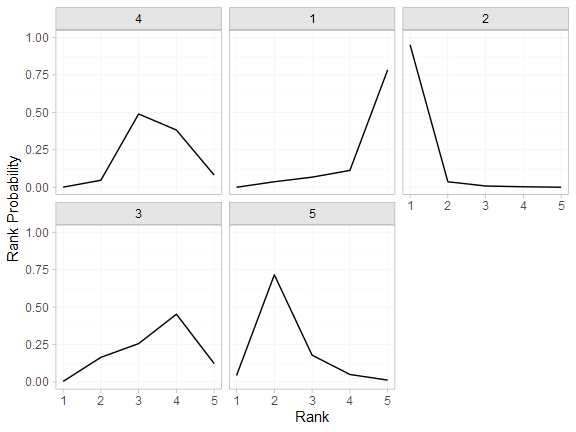(arm_cumrankprobs <- posterior_rank_probs(arm_fit_FE, cumulative = TRUE))
#>      p_rank p_rank p_rank p_rank p_rank
#> d      0.00      0.05      0.54      0.92         1
#> d      0.00      0.04      0.10      0.22         1
#> d      0.95      0.99      1.00      1.00         1
#> d      0.00      0.17      0.42      0.88         1
#> d      0.04      0.76      0.94      0.99         1
plot(arm_cumrankprobs)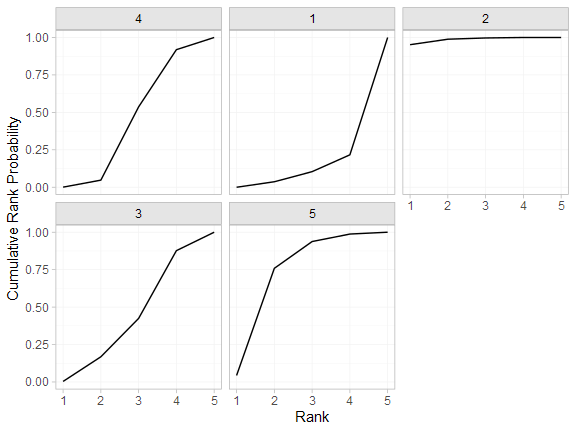## Analysis of contrast-based data

We now perform an analysis using the contrast-based data (mean differences and standard errors).

### Setting up the network

With contrast-level data giving the mean difference in off-time reduction (diff) and standard error (se_diff), we use the function set_agd_contrast() to set up the network.

contr_net <- set_agd_contrast(parkinsons,
study = studyn,
trt = trtn,
y = diff,
se = se_diff,
sample_size = n)
contr_net
#> A network with 7 AgD studies (contrast-based).
#>
#> -------------------------------------------------- AgD studies (contrast-based) ----
#>  Study Treatments
#>  1     2: 1 | 3
#>  2     2: 1 | 2
#>  3     3: 1 | 2 | 4
#>  4     2: 3 | 4
#>  5     2: 3 | 4
#>  6     2: 4 | 5
#>  7     2: 4 | 5
#>
#>  Outcome type: continuous
#> ------------------------------------------------------------------------------------
#> Total number of treatments: 5
#> Total number of studies: 7
#> Reference treatment is: 4
#> Network is connected

The sample_size argument is optional, but enables the nodes to be weighted by sample size in the network plot.

Plot the network structure.

plot(contr_net, weight_edges = TRUE, weight_nodes = TRUE)### Meta-analysis models

We fit both fixed effect (FE) and random effects (RE) models.

#### Fixed effect meta-analysis

First, we fit a fixed effect model using the nma() function with trt_effects = "fixed". We use $$\mathrm{N}(0, 100^2)$$ prior distributions for the treatment effects $$d_k$$. We can examine the range of parameter values implied by these prior distributions with the summary() method:

summary(normal(scale = 100))
#> A Normal prior distribution: location = 0, scale = 100.
#> 50% of the prior density lies between -67.45 and 67.45.
#> 95% of the prior density lies between -196 and 196.

The model is fitted using the nma() function.

contr_fit_FE <- nma(contr_net,
trt_effects = "fixed",
prior_trt = normal(scale = 100))
#> Note: Setting "4" as the network reference treatment.

Basic parameter summaries are given by the print() method:

contr_fit_FE
#> A fixed effects NMA with a normal likelihood (identity link).
#> Inference for Stan model: normal.
#> 4 chains, each with iter=2000; warmup=1000; thin=1;
#> post-warmup draws per chain=1000, total post-warmup draws=4000.
#>
#>       mean se_mean   sd  2.5%   25%   50%   75% 97.5% n_eff Rhat
#> d  0.52    0.01 0.48 -0.44  0.20  0.53  0.86  1.45  2111    1
#> d -1.27    0.01 0.53 -2.33 -1.63 -1.27 -0.90 -0.24  2086    1
#> d  0.05    0.01 0.33 -0.59 -0.17  0.05  0.27  0.69  3225    1
#> d -0.30    0.00 0.21 -0.71 -0.44 -0.29 -0.16  0.11  3830    1
#> lp__ -3.21    0.03 1.45 -7.03 -3.87 -2.86 -2.15 -1.40  1735    1
#>
#> Samples were drawn using NUTS(diag_e) at Tue Jun 23 15:50:19 2020.
#> For each parameter, n_eff is a crude measure of effective sample size,
#> and Rhat is the potential scale reduction factor on split chains (at
#> convergence, Rhat=1).

The prior and posterior distributions can be compared visually using the plot_prior_posterior() function:

plot_prior_posterior(contr_fit_FE)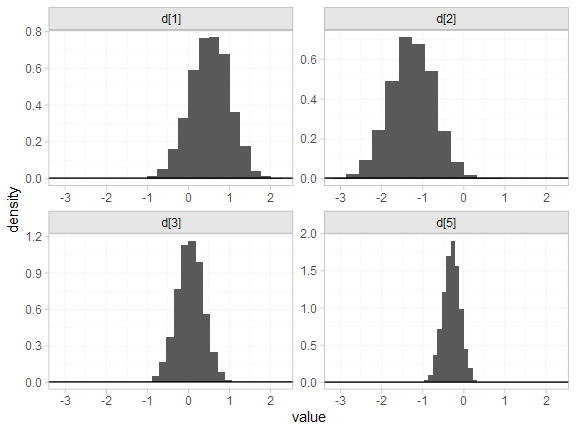#### Random effects meta-analysis

We now fit a random effects model using the nma() function with trt_effects = "random". Again, we use $$\mathrm{N}(0, 100^2)$$ prior distributions for the treatment effects $$d_k$$, and we additionally use a $$\textrm{half-N}(5^2)$$ prior for the heterogeneity standard deviation $$\tau$$. We can examine the range of parameter values implied by these prior distributions with the summary() method:

summary(normal(scale = 100))
#> A Normal prior distribution: location = 0, scale = 100.
#> 50% of the prior density lies between -67.45 and 67.45.
#> 95% of the prior density lies between -196 and 196.
summary(half_normal(scale = 5))
#> A half-Normal prior distribution: location = 0, scale = 5.
#> 50% of the prior density lies between 0 and 3.37.
#> 95% of the prior density lies between 0 and 9.8.

Fitting the RE model

contr_fit_RE <- nma(contr_net,
seed = 1150676438,
trt_effects = "random",
prior_trt = normal(scale = 100),
prior_het = half_normal(scale = 5),
#> Note: Setting "4" as the network reference treatment.
#> Warning: There were 3 transitions after warmup that exceeded the maximum treedepth. Increase max_treedepth above 10. See
#> http://mc-stan.org/misc/warnings.html#maximum-treedepth-exceeded
#> Warning: Examine the pairs() plot to diagnose sampling problems

We do see a small number of divergent transition errors, which cannot simply be removed by increasing the value of the adapt_delta argument (by default set to 0.95 for RE models). To diagnose, we use the pairs() method to investigate where in the posterior distribution these divergences are happening (indicated by red crosses):

pairs(contr_fit_RE, pars = c("d", "delta[4: 4 vs. 3]", "tau"))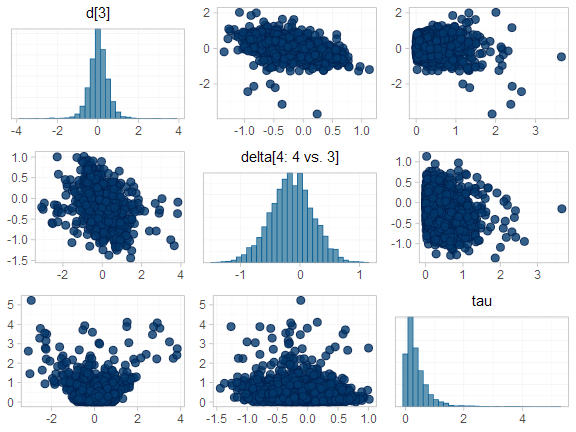The divergent transitions occur in the upper tail of the heterogeneity standard deviation. In this case, with only a small number of studies, there is not very much information to estimate the heterogeneity standard deviation and the prior distribution may be too heavy-tailed. We could consider a more informative prior distribution for the heterogeneity variance to aid estimation.

Basic parameter summaries are given by the print() method:

contr_fit_RE
#> A random effects NMA with a normal likelihood (identity link).
#> Inference for Stan model: normal.
#> 4 chains, each with iter=2000; warmup=1000; thin=1;
#> post-warmup draws per chain=1000, total post-warmup draws=4000.
#>
#>       mean se_mean   sd   2.5%   25%   50%   75% 97.5% n_eff Rhat
#> d  0.54    0.01 0.63  -0.61  0.15  0.55  0.92  1.76  1926    1
#> d -1.30    0.01 0.68  -2.62 -1.71 -1.29 -0.87  0.00  2134    1
#> d  0.04    0.01 0.51  -0.92 -0.23  0.03  0.31  0.97  1946    1
#> d -0.32    0.01 0.49  -1.20 -0.52 -0.31 -0.10  0.51  1199    1
#> lp__ -8.25    0.08 2.88 -14.72 -9.99 -7.97 -6.21 -3.38  1218    1
#> tau   0.40    0.02 0.44   0.01  0.12  0.27  0.52  1.46   547    1
#>
#> Samples were drawn using NUTS(diag_e) at Tue Jun 23 15:50:42 2020.
#> For each parameter, n_eff is a crude measure of effective sample size,
#> and Rhat is the potential scale reduction factor on split chains (at
#> convergence, Rhat=1).

By default, summaries of the study-specific relative effects $$\delta_{jk}$$ are hidden, but could be examined by changing the pars argument:

# Not run
print(contr_fit_RE, pars = c("d", "delta"))

The prior and posterior distributions can be compared visually using the plot_prior_posterior() function:

plot_prior_posterior(contr_fit_RE)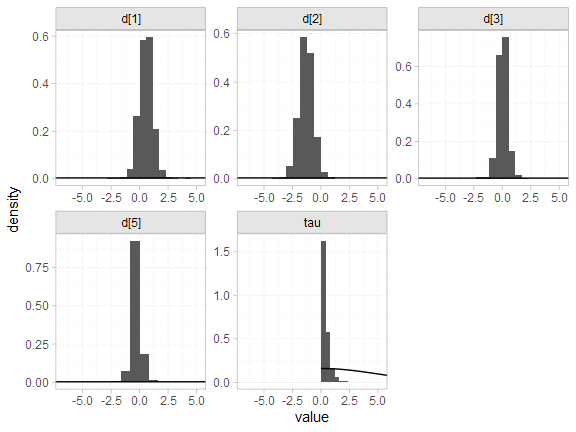#### Model comparison

Model fit can be checked using the dic() function:

(contr_dic_FE <- dic(contr_fit_FE))
#> Residual deviance: 6.4 (on 8 data points)
#>                pD: 4.1
#>               DIC: 10.5
(contr_dic_RE <- dic(contr_fit_RE))
#> Residual deviance: 6.6 (on 8 data points)
#>                pD: 5.4
#>               DIC: 11.9

Both models fit the data well, having posterior mean residual deviance close to the number of data points. The DIC is similar between models, so we choose the FE model based on parsimony.

We can also examine the residual deviance contributions with the corresponding plot() method.

plot(contr_dic_FE)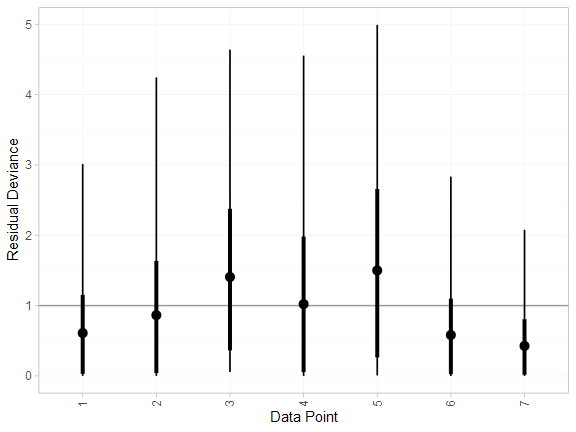plot(contr_dic_RE)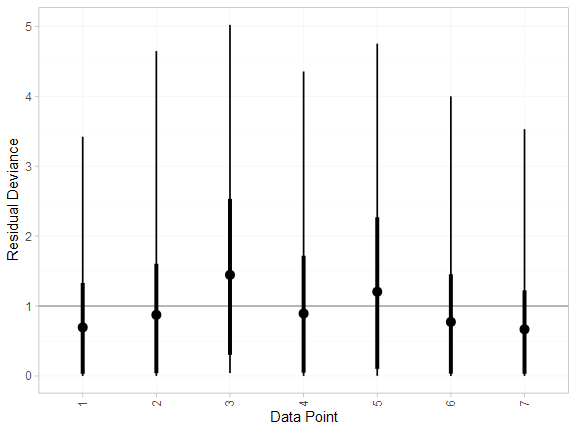### Further results

For comparison with Dias et al. (2011), we can produce relative effects against placebo using the relative_effects() function with trt_ref = 1:

(contr_releff_FE <- relative_effects(contr_fit_FE, trt_ref = 1))
#>       mean   sd  2.5%   25%   50%   75% 97.5% Bulk_ESS Tail_ESS Rhat
#> d -0.52 0.48 -1.45 -0.86 -0.53 -0.20  0.44     2119     2437    1
#> d -1.80 0.34 -2.45 -2.02 -1.81 -1.57 -1.13     5039     2667    1
#> d -0.48 0.49 -1.44 -0.81 -0.49 -0.15  0.49     3014     2848    1
#> d -0.82 0.53 -1.82 -1.19 -0.83 -0.47  0.23     2191     2602    1
plot(contr_releff_FE, ref_line = 0)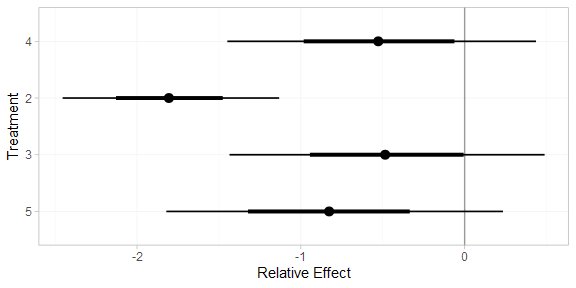(contr_releff_RE <- relative_effects(contr_fit_RE, trt_ref = 1))
#>       mean   sd  2.5%   25%   50%   75% 97.5% Bulk_ESS Tail_ESS Rhat
#> d -0.54 0.63 -1.76 -0.92 -0.55 -0.15  0.61     2138     1924    1
#> d -1.85 0.51 -2.90 -2.14 -1.83 -1.55 -0.84     3331     1931    1
#> d -0.51 0.64 -1.77 -0.89 -0.51 -0.11  0.73     3047     2375    1
#> d -0.86 0.77 -2.33 -1.30 -0.85 -0.41  0.56     2109     1383    1
plot(contr_releff_RE, ref_line = 0)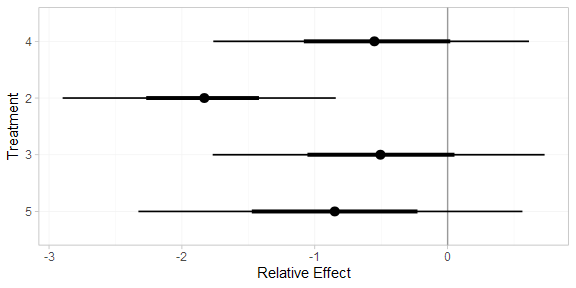Following Dias et al. (2011), we produce absolute predictions of the mean off-time reduction on each treatment assuming a Normal distribution for the outcomes on treatment 1 (placebo) with mean $$-0.73$$ and precision $$21$$. We use the predict() method, where the baseline argument takes a distr() distribution object with which we specify the corresponding Normal distribution, and we specify trt_ref = 1 to indicate that the baseline distribution corresponds to treatment 1. (Strictly speaking, type = "response" is unnecessary here, since the identity link function was used.)

contr_pred_FE <- predict(contr_fit_FE,
baseline = distr(qnorm, mean = -0.73, sd = 21^-0.5),
type = "response",
trt_ref = 1)
contr_pred_FE
#>          mean   sd  2.5%   25%   50%   75% 97.5% Bulk_ESS Tail_ESS Rhat
#> pred -1.25 0.53 -2.26 -1.61 -1.26 -0.91 -0.20     2418     2939    1
#> pred -0.73 0.22 -1.16 -0.88 -0.73 -0.58 -0.30     3967     3603    1
#> pred -2.53 0.41 -3.32 -2.80 -2.53 -2.26 -1.72     4581     3473    1
#> pred -1.21 0.53 -2.27 -1.56 -1.21 -0.86 -0.14     3238     3296    1
#> pred -1.55 0.57 -2.66 -1.94 -1.55 -1.17 -0.43     2442     2593    1
plot(contr_pred_FE)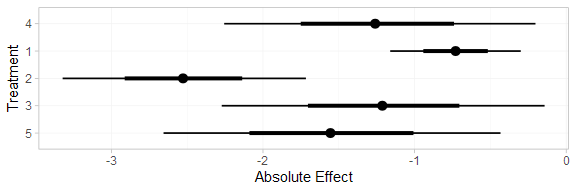contr_pred_RE <- predict(contr_fit_RE,
baseline = distr(qnorm, mean = -0.73, sd = 21^-0.5),
type = "response",
trt_ref = 1)
contr_pred_RE
#>          mean   sd  2.5%   25%   50%   75% 97.5% Bulk_ESS Tail_ESS Rhat
#> pred -1.28 0.67 -2.57 -1.69 -1.27 -0.86 -0.01     2310     2013    1
#> pred -0.73 0.22 -1.16 -0.88 -0.73 -0.59 -0.30     3676     3583    1
#> pred -2.58 0.55 -3.71 -2.91 -2.57 -2.24 -1.53     3522     2588    1
#> pred -1.24 0.68 -2.57 -1.65 -1.24 -0.83  0.12     3154     2500    1
#> pred -1.60 0.80 -3.16 -2.06 -1.57 -1.12 -0.15     2229     1559    1
plot(contr_pred_RE)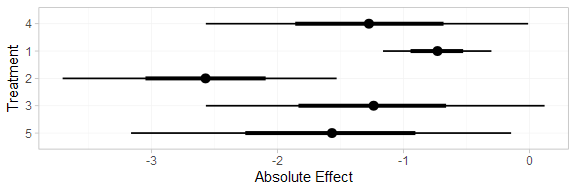If the baseline argument is omitted an error will be raised, as there are no study baselines estimated in this network.

# Not run
predict(contr_fit_FE, type = "response")

We can also produce treatment rankings, rank probabilities, and cumulative rank probabilities.

(contr_ranks <- posterior_ranks(contr_fit_FE))
#>         mean   sd 2.5% 25% 50% 75% 97.5% Bulk_ESS Tail_ESS Rhat
#> rank 3.51 0.71    2   3   3   4     5     2525       NA    1
#> rank 4.63 0.78    2   5   5   5     5     2661       NA    1
#> rank 1.05 0.29    1   1   1   1     2     2224     2316    1
#> rank 3.52 0.94    2   3   4   4     5     3636       NA    1
#> rank 2.29 0.67    1   2   2   3     4     2736     3007    1
plot(contr_ranks)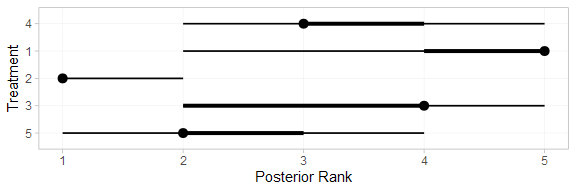(contr_rankprobs <- posterior_rank_probs(contr_fit_FE))
#>      p_rank p_rank p_rank p_rank p_rank
#> d      0.00      0.04      0.49      0.38      0.08
#> d      0.00      0.04      0.06      0.11      0.78
#> d      0.96      0.03      0.01      0.00      0.00
#> d      0.00      0.18      0.24      0.45      0.12
#> d      0.04      0.71      0.19      0.05      0.01
plot(contr_rankprobs)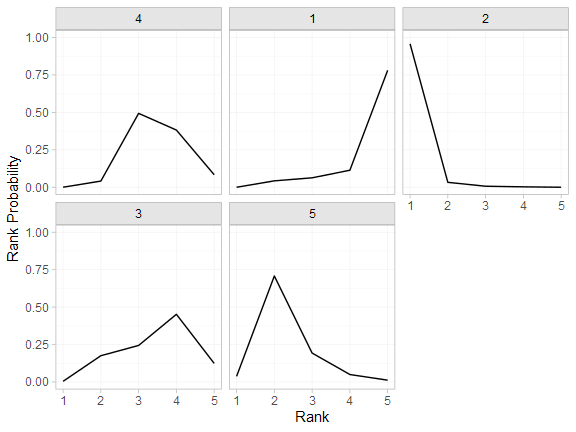(contr_cumrankprobs <- posterior_rank_probs(contr_fit_FE, cumulative = TRUE))
#>      p_rank p_rank p_rank p_rank p_rank
#> d      0.00      0.04      0.53      0.92         1
#> d      0.00      0.04      0.11      0.22         1
#> d      0.96      0.99      1.00      1.00         1
#> d      0.00      0.18      0.42      0.88         1
#> d      0.04      0.75      0.94      0.99         1
plot(contr_cumrankprobs)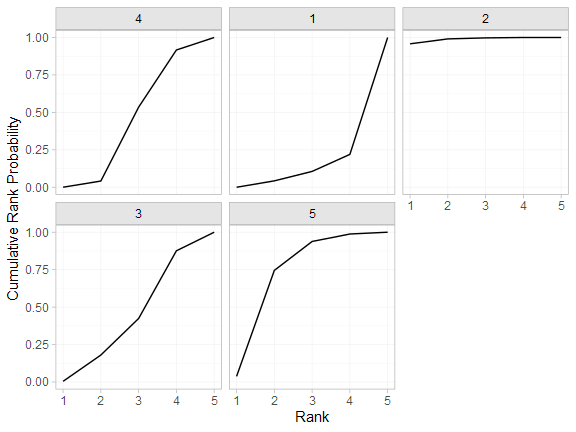## Analysis of mixed arm-based and contrast-based data

We now perform an analysis where some studies contribute arm-based data, and other contribute contrast-based data. Replicating Dias et al. (2011), we consider arm-based data from studies 1-3, and contrast-based data from studies 4-7.

studies <- parkinsons\$studyn
(parkinsons_arm <- parkinsons[studies %in% 1:3, ])
#>   studyn trtn     y    se   n  diff se_diff
#> 1      1    1 -1.22 0.504  54    NA   0.504
#> 2      1    3 -1.53 0.439  95 -0.31   0.668
#> 3      2    1 -0.70 0.282 172    NA   0.282
#> 4      2    2 -2.40 0.258 173 -1.70   0.382
#> 5      3    1 -0.30 0.505  76    NA   0.505
#> 6      3    2 -2.60 0.510  71 -2.30   0.718
#> 7      3    4 -1.20 0.478  81 -0.90   0.695
(parkinsons_contr <- parkinsons[studies %in% 4:7, ])
#>    studyn trtn     y    se   n  diff se_diff
#> 8       4    3 -0.24 0.265 128    NA   0.265
#> 9       4    4 -0.59 0.354  72 -0.35   0.442
#> 10      5    3 -0.73 0.335  80    NA   0.335
#> 11      5    4 -0.18 0.442  46  0.55   0.555
#> 12      6    4 -2.20 0.197 137    NA   0.197
#> 13      6    5 -2.50 0.190 131 -0.30   0.274
#> 14      7    4 -1.80 0.200 154    NA   0.200
#> 15      7    5 -2.10 0.250 143 -0.30   0.320

### Setting up the network

We use the functions set_agd_arm() and set_agd_contrast() to set up the respective data sources within the network, and then combine together with combine_network().

mix_arm_net <- set_agd_arm(parkinsons_arm,
study = studyn,
trt = trtn,
y = y,
se = se,
sample_size = n)

mix_contr_net <- set_agd_contrast(parkinsons_contr,
study = studyn,
trt = trtn,
y = diff,
se = se_diff,
sample_size = n)

mix_net <- combine_network(mix_arm_net, mix_contr_net)
mix_net
#> A network with 3 AgD studies (arm-based), and 4 AgD studies (contrast-based).
#>
#> ------------------------------------------------------- AgD studies (arm-based) ----
#>  Study Treatments
#>  1     2: 1 | 3
#>  2     2: 1 | 2
#>  3     3: 1 | 2 | 4
#>
#>  Outcome type: continuous
#> -------------------------------------------------- AgD studies (contrast-based) ----
#>  Study Treatments
#>  4     2: 3 | 4
#>  5     2: 3 | 4
#>  6     2: 4 | 5
#>  7     2: 4 | 5
#>
#>  Outcome type: continuous
#> ------------------------------------------------------------------------------------
#> Total number of treatments: 5
#> Total number of studies: 7
#> Reference treatment is: 4
#> Network is connected

The sample_size argument is optional, but enables the nodes to be weighted by sample size in the network plot.

Plot the network structure.

plot(mix_net, weight_edges = TRUE, weight_nodes = TRUE)### Meta-analysis models

We fit both fixed effect (FE) and random effects (RE) models.

#### Fixed effect meta-analysis

First, we fit a fixed effect model using the nma() function with trt_effects = "fixed". We use $$\mathrm{N}(0, 100^2)$$ prior distributions for the treatment effects $$d_k$$ and study-specific intercepts $$\mu_j$$. We can examine the range of parameter values implied by these prior distributions with the summary() method:

summary(normal(scale = 100))
#> A Normal prior distribution: location = 0, scale = 100.
#> 50% of the prior density lies between -67.45 and 67.45.
#> 95% of the prior density lies between -196 and 196.

The model is fitted using the nma() function.

mix_fit_FE <- nma(mix_net,
trt_effects = "fixed",
prior_intercept = normal(scale = 100),
prior_trt = normal(scale = 100))
#> Note: Setting "4" as the network reference treatment.

Basic parameter summaries are given by the print() method:

mix_fit_FE
#> A fixed effects NMA with a normal likelihood (identity link).
#> Inference for Stan model: normal.
#> 4 chains, each with iter=2000; warmup=1000; thin=1;
#> post-warmup draws per chain=1000, total post-warmup draws=4000.
#>
#>       mean se_mean   sd  2.5%   25%   50%   75% 97.5% n_eff Rhat
#> d  0.51    0.01 0.48 -0.42  0.19  0.52  0.85  1.44  1364    1
#> d -1.30    0.01 0.52 -2.36 -1.65 -1.30 -0.95 -0.29  1403    1
#> d  0.04    0.01 0.32 -0.57 -0.18  0.04  0.26  0.69  2293    1
#> d -0.30    0.00 0.21 -0.70 -0.44 -0.29 -0.16  0.11  3114    1
#> lp__ -4.62    0.05 1.87 -9.16 -5.64 -4.28 -3.24 -1.98  1490    1
#>
#> Samples were drawn using NUTS(diag_e) at Tue Jun 23 15:51:13 2020.
#> For each parameter, n_eff is a crude measure of effective sample size,
#> and Rhat is the potential scale reduction factor on split chains (at
#> convergence, Rhat=1).

By default, summaries of the study-specific intercepts $$\mu_j$$ are hidden, but could be examined by changing the pars argument:

# Not run
print(mix_fit_FE, pars = c("d", "mu"))

The prior and posterior distributions can be compared visually using the plot_prior_posterior() function:

plot_prior_posterior(mix_fit_FE)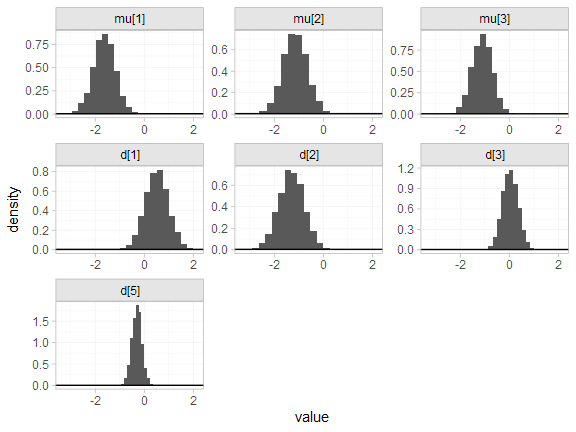#### Random effects meta-analysis

We now fit a random effects model using the nma() function with trt_effects = "random". Again, we use $$\mathrm{N}(0, 100^2)$$ prior distributions for the treatment effects $$d_k$$ and study-specific intercepts $$\mu_j$$, and we additionally use a $$\textrm{half-N}(5^2)$$ prior for the heterogeneity standard deviation $$\tau$$. We can examine the range of parameter values implied by these prior distributions with the summary() method:

summary(normal(scale = 100))
#> A Normal prior distribution: location = 0, scale = 100.
#> 50% of the prior density lies between -67.45 and 67.45.
#> 95% of the prior density lies between -196 and 196.
summary(half_normal(scale = 5))
#> A half-Normal prior distribution: location = 0, scale = 5.
#> 50% of the prior density lies between 0 and 3.37.
#> 95% of the prior density lies between 0 and 9.8.

Fitting the RE model

mix_fit_RE <- nma(mix_net,
seed = 437219664,
trt_effects = "random",
prior_intercept = normal(scale = 100),
prior_trt = normal(scale = 100),
prior_het = half_normal(scale = 5),
#> Warning: Examine the pairs() plot to diagnose sampling problems
We do see a small number of divergent transition errors, which cannot simply be removed by increasing the value of the adapt_delta argument (by default set to 0.95 for RE models). To diagnose, we use the pairs() method to investigate where in the posterior distribution these divergences are happening (indicated by red crosses):
pairs(mix_fit_RE, pars = c("d", "delta[4: 4 vs. 3]", "tau"))